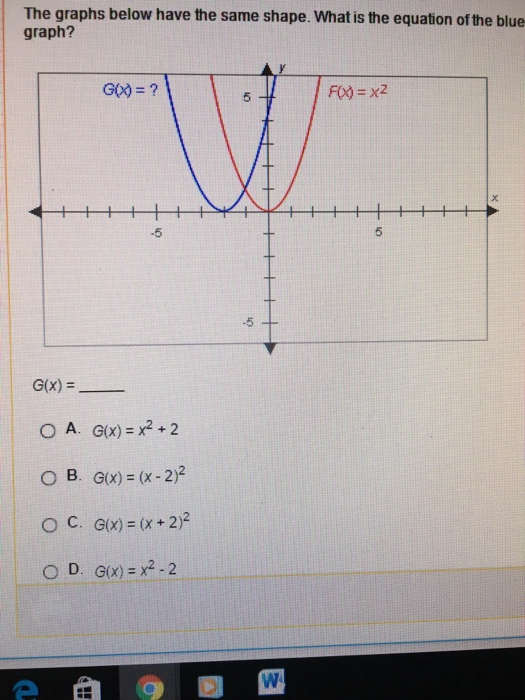# The graphs below have the same shape what is the equation of the blue graphThe graphs below have the same shape. What is the equation of the blue graph? G(x) = A. G(x) = x^2 _+ 2 B. B. G(x) = (x – 2)^2 C. G(x) = (x + 2)^2 D. G(x) = x^2 – 2

Quein The graßhe below have the same shape. is the equation of the blue graph? what ‘F(x) = x² (+2)2 4 2 – – Vo7 -2 -1 2 Soler first recall some facts is Consider a function f(x), Define new function gex) = f(xrth), where h be Constant. a then for ‘the value of the graph will shift sight, and for we value of h, großh will shift left,
here, the given function is Fle) = ? It is clean from the graph of frow, it touches origin (cie at 2:o). it x.am’s at M. Aris at x-2, Also, the new function Gius, touches {by graph of Gre). graph of free) and Geus have the same shape. graph of fex is shifted 2 units to the left. h = -2 & ) quation of Gen) is f (x-(-3)) {uning a bone facts. f (x+2) funda) * {: fixe :x² quation of Gile) (22+2) Awe ” option cey is Comect,